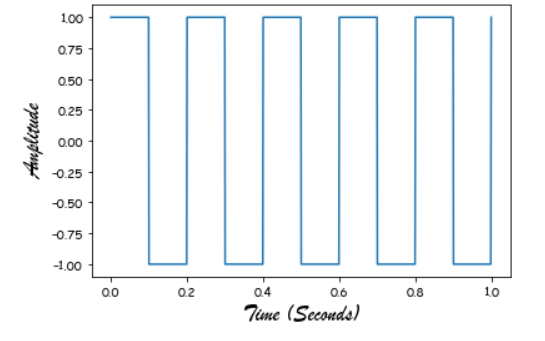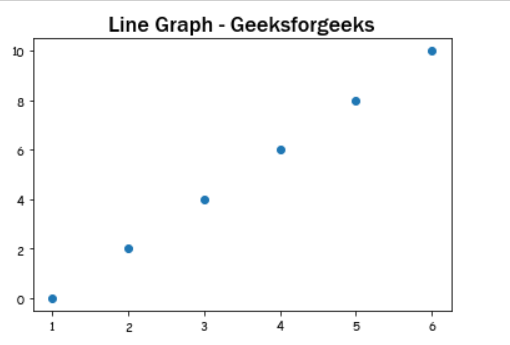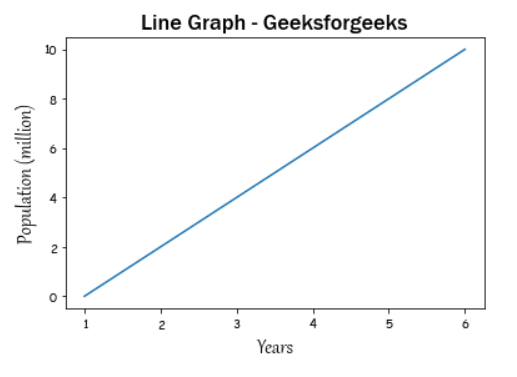# How to Change Fonts in matplotlib?

Prerequisites:  Matplotlib

In this article, we will see how can we can change the font family of our graph using matplotlib. A variety of fonts are in fact supported by matplotlib, alone has to do in order to implement is pass the name as value to fontname parameter.

Approach:

• Import required library.
• Create data
• Set changed font
• Normally plot the data
• Display plot

Example 1: Change the font just for the axis labels.

## Python3

 `from` `scipy ``import` `signal ` `import` `matplotlib.pyplot as plot ` `import` `numpy as np ` `# %matplotlib inline ` ` `  `# Plot the square wave ` `t ``=` `np.linspace(``0``, ``1``, ``1000``, endpoint``=``True``) ` `plot.plot(t, signal.square(``2` `*` `np.pi ``*` `5` `*` `t)) ` ` `  `# Change the x, y axis label to "Brush Script MT" font style. ` `plot.xlabel(``"Time (Seconds)"``, fontname``=``"Brush Script MT"``, fontsize``=``18``) ` `plot.ylabel(``"Amplitude"``, fontname``=``"Brush Script MT"``, fontsize``=``18``) ` ` `  `plot.show() `

Output:Example 2: Change the font just for the title.

## Python3

 `import` `matplotlib.pyplot as plot ` ` `  `x ``=` `[``1``, ``2``, ``3``, ``4``, ``5``, ``6``] ` `y ``=` `[``0``, ``2``, ``4``, ``6``, ``8``, ``10``] ` ` `  `# plotting a plot ` `plot.scatter(x, y) ` ` `  `# Set the title to 'Franklin Gothic Medium' style. ` `plot.title(``"Line Graph - Geeksforgeeks"``, ` `           ``fontname``=``'Franklin Gothic Medium'``, fontsize``=``18``) ` ` `  `plot.show() `

Output:Example 3: Change the font just for the title and axis labels.

## Python3

 `import` `matplotlib.pyplot as plot ` ` `  `x ``=` `[``1``, ``2``, ``3``, ``4``, ``5``, ``6``] ` `y ``=` `[``0``, ``2``, ``4``, ``6``, ``8``, ``10``] ` ` `  `# plotting a plot ` `plot.plot(x, y) ` ` `  ` `  `# Change the x, y axis label to 'Gabriola' style. ` `plot.xlabel(``"Years"``, fontname``=``"Gabriola"``, fontsize``=``18``) ` `plot.ylabel(``"Population (million)"``, fontname``=``"Gabriola"``, fontsize``=``18``) ` ` `  `# Set the title to 'Franklin Gothic Medium' style. ` `plot.title(``"Line Graph - Geeksforgeeks"``, ` `           ``fontname``=``'Franklin Gothic Medium'``, fontsize``=``18``) ` ` `  `plot.show() `

Output:Whether you're preparing for your first job interview or aiming to upskill in this ever-evolving tech landscape, GeeksforGeeks Courses are your key to success. We provide top-quality content at affordable prices, all geared towards accelerating your growth in a time-bound manner. Join the millions we've already empowered, and we're here to do the same for you. Don't miss out - check it out now!

Previous
Next